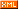# Idiot-maker

:: :: :: :: :: :::: ::291 随笔 :: 0 文章 :: 20 评论 :: 0 引用

https://leetcode.com/problems/two-sum-ii-input-array-is-sorted/

Given an array of integers that is already sorted in ascending order, find two numbers such that they add up to a specific target number.

The function twoSum should return indices of the two numbers such that they add up to the target, where index1 must be less than index2.

Note:

• Your returned answers (both index1 and index2) are not zero-based.
• You may assume that each input would have exactly one solution and you may not use the same element twice.

Example:

Input: numbers = [2,7,11,15], target = 9
Output: [1,2]
Explanation: The sum of 2 and 7 is 9. Therefore index1 = 1, index2 = 2.

class Solution {
public int[] twoSum(int[] numbers, int target) {
int start = 0, end = numbers.length - 1;
int[] res = new int;
while (start < end) {
if (numbers[start] + numbers[end] == target) {
res = start + 1;
res = end + 1;
return res;
} else if (numbers[start] + numbers[end] < target) {
start++;
} else {
end--;
}
}
return res;
}
}

Given an array of integers that is already sorted in ascending order, find two numbers such that they add up to a specific target number.

The function twoSum should return indices of the two numbers such that they add up to the target, where index1 must be less than index2.

Note:

• Your returned answers (both index1 and index2) are not zero-based.
• You may assume that each input would have exactly one solution and you may not use the same element twice.

Example:

Input: numbers = [2,7,11,15], target = 9
Output: [1,2]
Explanation: The sum of 2 and 7 is 9. Therefore index1 = 1, index2 = 2.
posted on 2018-12-24 23:15 NickyYe 阅读(...) 评论(...) 编辑 收藏